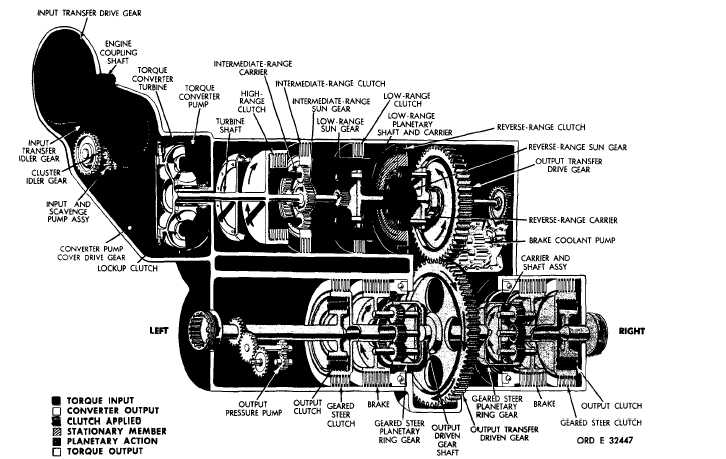Figure   28.   Reverse   2-gear   torque   path,   converter   operation
Custom SearchPAR   5  2 T O R Q U E     P A T HS C H A P    2,   SEC  V Figure   28.   Reverse   2-gear   torque   path,   converter   operation 52.   TORQUE   PATH   -   REVERSE   2   GEAR, output   transfer   drive   gear   as   described   in   par. CONVERTER   OPERATION   (fig.   28) 51c   and   ,   above.   The   remainder   of   the   torque path   is   identical   to   that   described   in   par.   48, Torque   is   transmitted   to   the   turbine   shaft above,   for   second   gear.   Refer   to   TABULATED as   described   in   pars.   42   and   43,   above.   Torque D A T A ,    p a r .    7 ,    f o r    g e a r    r a t i o s    a p p l i c a b l e    to is   transmitted   through   the   range   gearing   to   the r e v e r s e    2    g e a r. 5 5Integrated Publishing, Inc. - A (SDVOSB) Service Disabled Veteran Owned Small Business